《点睛：ActionScript3.0游戏互动编程》——1.2　ColorTransform对RGB数值的操作及应用

0
0
01. 云栖社区>
2. 博客>
3. 正文

《点睛：ActionScript3.0游戏互动编程》——1.2　ColorTransform对RGB数值的操作及应用

1.2　ColorTransform对RGB数值的操作及应用

flash.geom.ColorTransform是Flash内置的一个色彩变换类。它支持色彩通道值的线性变换。

red(dst) = red(src) * redMultiplier + redOffset
green(dst) = green(src) * greenMultiplier + greenOffset
blue(dst) = blue(src) * blueMultiplier + blueOffset
alpha(dst) = alpha(src) * alphaMultiplier + alphaOffset

myColorTransform.redMultiplier = myColorTransform.greenMultiplier = myColorTransform.blueMultipler = 0;
myColorTransform.redOffset = 255;
myColorTransform.greenOffset = 255;
myColorTransform.blueOffset = 0;

ColorTransform最常用的地方有两处。

1 DisplayObject.transform.colorTransform = myColorTransform;
2 BitmapData.colorTransform(myColorTransform);

1.2.1　RGB测试用例的书写

package{
[SWF(width = "800", height = "600")]
public class ShapeColorTransformTest extends Sprite{
private var _testSprite_src:Sprite; //用于测试的显示对象（变换前）
private var _testSprite_dst:Sprite;  //用于测试的显示对象（变换后）
private var _myColorTransform:ColorTransform;  //颜色转换对象
public function ShapeColorTransformTest(){
init();
}
private function init():void{
_testSprite_src = getTestSprite();
_testSprite_dst = getTestSprite();
_testSprite_dst.x = 200;
applyTransform();
}
//创建用于测试的显示对象
private function getTestSprite():Sprite{
var _testSprite:Sprite = new Sprite();
var _shape1:Shape = new Shape();  //添加一个黑色的矩形底
_shape1.graphics.beginFill(0x000000);
_shape1.graphics.drawRect(40, 30, 170, 290);
_shape1.graphics.endFill();
/*依次添加颜色分别为暗蓝、暗红、暗绿和纯白的4个圆*/
return _testSprite;
}
private function applyTransform():void{
_myColorTransform = _testSprite_dst.transform.colorTransform; //初始化颜色变换对象（从显示对象里获取）
_myColorTransform.redMultiplier = 1.5;
_testSprite_dst.transform.colorTransform = _myColorTransform; //应用到显示对象上（变换后必须重新赋值，否则变换效果不起作用，详情可查阅帮助文档）
}
}

package com.gemei.display{
public class Circle extends Sprite{
//为节省本书篇幅，我把x，y，alpha, blendMode这些基本属性都封装到Circle类里面了，项目开发中不推荐这么做
public function Circle(radius:Number = 50, color:uint = 0x000000, x:Number = 0, y:Number = 0, alpha:Number = 1, blendMode:String = BlendMode.NORMAL){
super();
this.x = x; this.y = y; this.blendMode = blendMode;
graphics.beginFill(color, alpha);
graphics.endFill();
}
}
}

1.2.2　初始效果及颜色属性的测试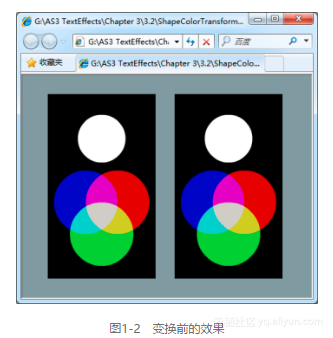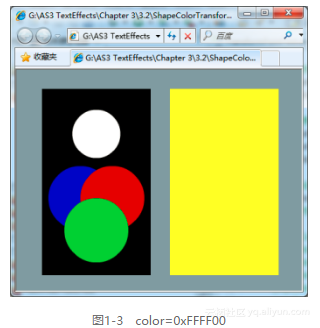_myColorTransform.redMultiplier = _myTransform.greenMultiplier = _myColorTransform.blueMultiplier = 0;
_myColorTransform.redOffset = _myColorTransform.greenOffset = 0xFF;

1.2.3　线性/倍乘提高降低亮度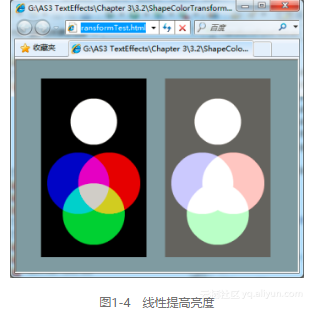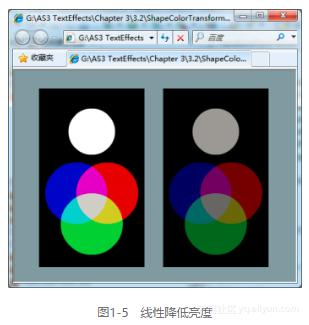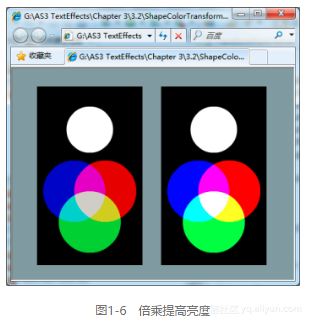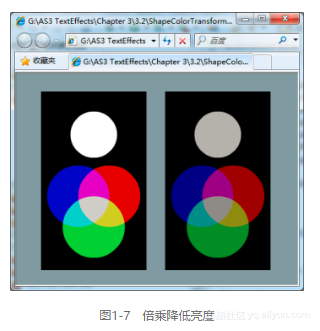_myColorTransform.redMultiplier = _myColorTransform.greenMultiplier = _myColorTransform. blueMultiplier = 1.5;
_myColorTransform.redOffset = _myColorTransform.greenOffset = _myColorTransform.blueOffset = - 127;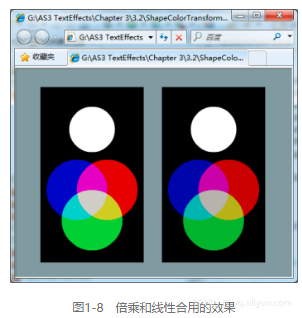1.2.4　单个通道的线性/倍乘变化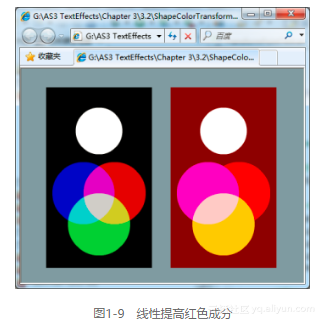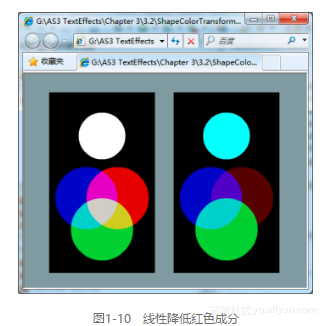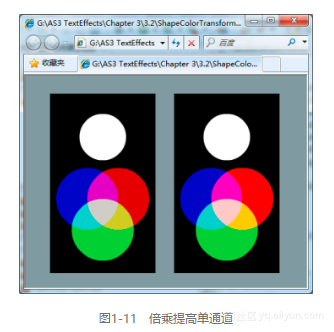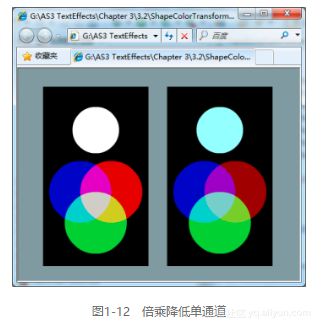1.2.5　ColorTransform在色彩处理方面的不足

**
1.2.6　Alpha测试用例的书写**

//创建用于测试的显示对象
private function getTestSprite():Sprite{
var _f:Array = [new BlurFilter(80, 80)];
var _testSprite:Sprite = new Sprite();
/*添加两个圆，颜色分别为白和黑*/
_testSprite.addChild(new Circle(50, 0xFFFFFF, 100, 100)).filters = _f;
_testSprite.addChild(new Circle(50, 0xFFFFFF, 100, 250)).filters = _f;
return _testSprite;
}

private function applyTransform():void{
_myColorTransform = _testSprite_dst.transform.colorTransform;
_myColorTransform.alphaMultiplier = 0;
_myColorTransform.alphaOffset = 127;
_testSprite_dst.transform.colorTransform = _myColorTransform;
}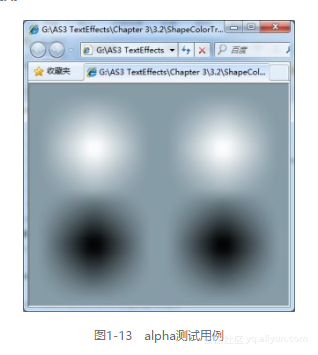**
1.2.7　线性提高或降低alpha值**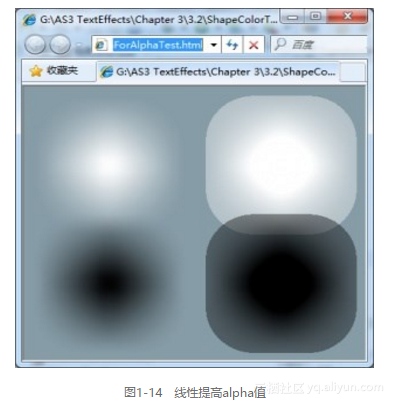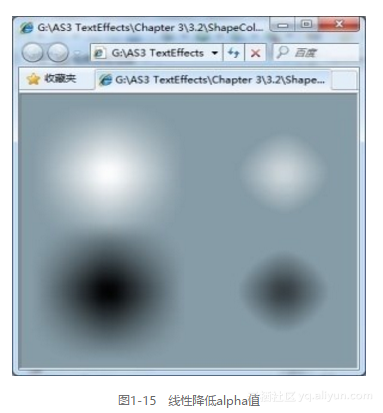1.2.8　设置alpha值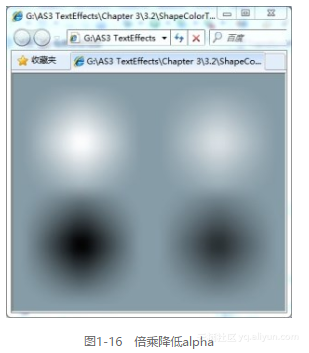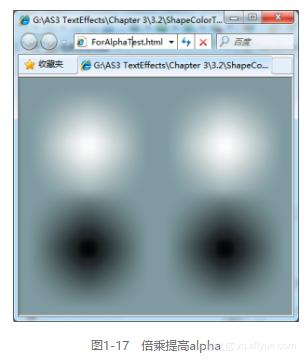_myColorTransform.alphaMultiplier = 0;
_myColorTransform.alphaOffset = 127;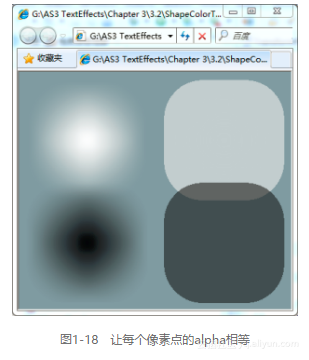1.2.9　用ColorTransform实现反色效果

dst = 255 – src;

red(dst) = red(src) * (-1) + 255。
green(dst) = green(src) * (-1) + 255
blue(dst) = blue(src) * (-1) + 255

_myColorTransform.redMultiplier = -1;
_myColorTransform.greenMultiplier =-1;
_myColorTransform.blueMultiplier = -1;
_myColorTransform.redOffset = 255;
_myColorTransform.greenOffset = 255;
_myColorTransform.blueOffset = 255;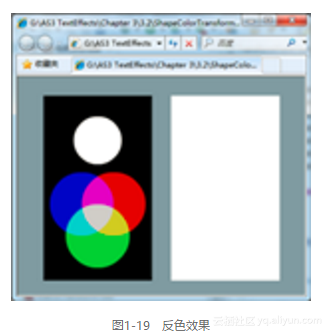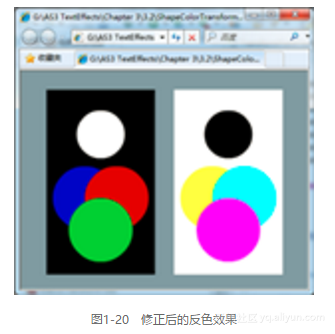+ 关注

corcosa 12572人浏览